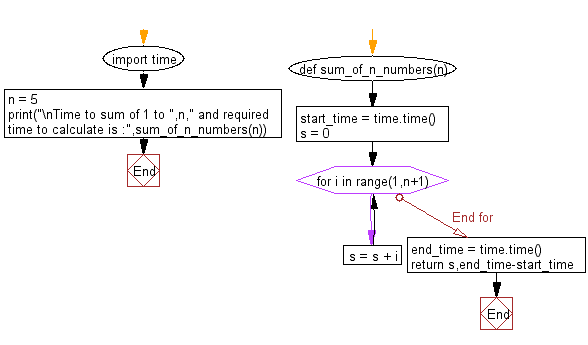﻿ Python: Get execution time - w3resource# Python: Get execution time

## Python Basic: Exercise-57 with Solution

Write a Python program to get execution time (in seconds) for a Python method.

Sample Solution:-

Python Code:

``````import time
def sum_of_n_numbers(n):
start_time = time.time()
s = 0
for i in range(1,n+1):
s = s + i
end_time = time.time()
return s,end_time-start_time

n = 5
print("\nTime to sum of 1 to ",n," and required time to calculate is :",sum_of_n_numbers(n))
```
```

Sample Output:

```Time to sum of 1 to  5  and required time to calculate is : (15, 2.384185791015625e-06)
```

Flowchart:## Visualize Python code execution:

The following tool visualize what the computer is doing step-by-step as it executes the said program:

Python Code Editor:

Have another way to solve this solution? Contribute your code (and comments) through Disqus.

What is the difficulty level of this exercise?

Test your Python skills with w3resource's quiz

﻿

## Python: Tips of the Day

Unpack variables from iterable:

```# One can unpack all iterables (tuples, list etc)
>>> a, b, c = 1, 2, 3
>>> a, b, c
(1, 2, 3)

>>> a, b, c = [1, 2, 3]
>>> a, b, c
(1, 2, 3)
```